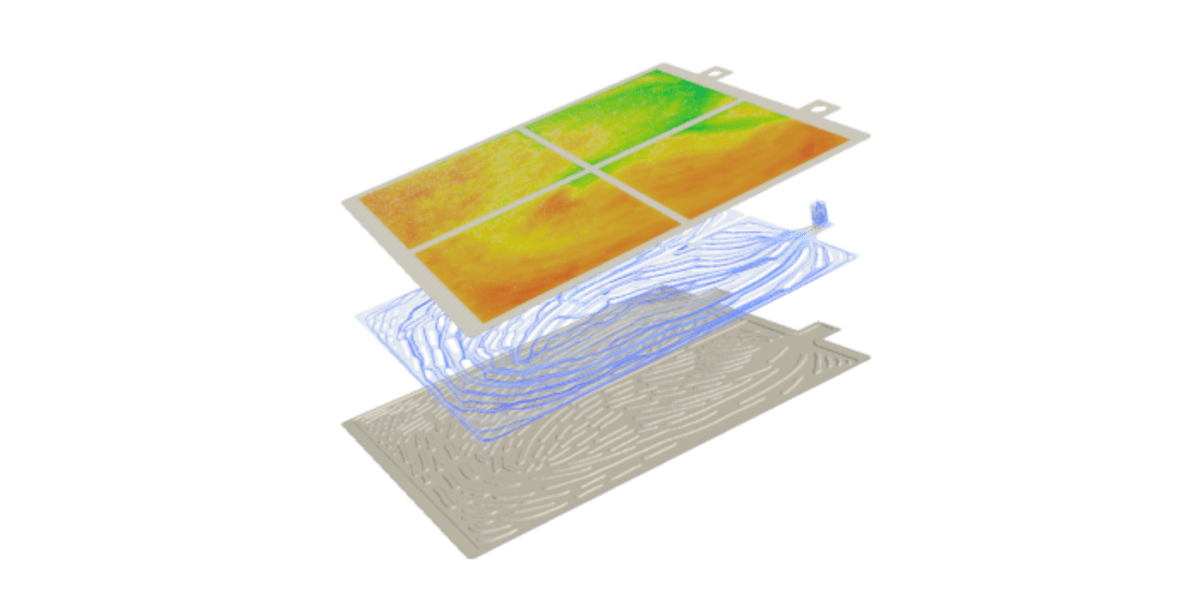Blog

News

## Multi-objective Optimization To Reduce Temperature | Diabatix

November 13, 2022byThere exist a wide range of types of optimization problems. Still, they can all be summarized as a combination of one or more objective functions subject to several additional constraints.

When more than one objective function is part of the optimizationproblem, this is called multi-objective optimization. The problem can be formulated as follows:

$\underset{x \in X}{\min} (J_1, J_2, ..., J_k)$

s.t.

$g_i(x) \leqq 0$

$h_j(x) = 0$

where $$J_k$$ are the objective functions, X is the solution space, $$g_i(x)$$ is the inequality constaints and $$h_j(x)$$ is the equality constraint functions.

One of the classic methods for multi-objective optimization is the weighted sum method. By multiplying each objective by a user-specified weight,the series of objectives turn into a single objective problem:

$\min w_1 J_1 + w_2 J_2 + ... = \sum w_i J_i$

where $$w_i$$ are the weighting factors and $$J_i$$ are the objective functions.

A weighting value allows the user to specify the importance of each objective. After selecting the desired objective, the user can change the weighting factor of it. A value equal to 1 translates to that objective having the highest priority, while a value close to 0 translates to the target having the lowest priority.

Constraints, on the other hand, must be satisfied as long as this is physically possible. The satisfaction of constraint(s) will always prioritize minimizing/maximizing objectives.

Taking advantage of the most recent webinar, Multi-objective optimization for battery cold plates, we will navigate into the three possible options to tackle a battery cold plate optimization on ColdStream.

#### 1. Single objective optimization with temperature minimization and fixed value pressure constraints plus manufacturing constraint

Temperature minimization should be used if the aim is to reduce the temperature across a component by focusing on the highest temperatures. Lowering the highest temperatures on a component results in a decrease in the mean temperature. Furthermore, the location of the highest temperature in a component may also change. The mathematical formulation involves integrating the temperature over a surface or volume:

$\min \int T \mathrm{d} s$

s.t.

$\Delta T < 6 ^\circ C$

$\Delta p < 30 \mathrm{kPa}$

$\mathrm{Manufacturing}$

where the optimization problem is subjected to a constraint of temperature variation smaller than $$6 ^\circ C$$, a maximum pressure variation of 30 kPa and the manufacturing constraint for the sheet metal forming.

#### 2. Multi-objective optimization with temperature minimization, temperature variance minimization and fixed value pressure constraints plus manufacturing constraint

Apart from temperature minimization, another target is considered for this case: temperature variance minimization. In this manner, the case turns into a multi-objective optimization, incorporating the weighted sum method into the objective.

The temperature variance minimization reduces the temperature fluctuations across a component and makes the temperature spread as uniform as possible. This can be especially important for thermal stresses. In other words, the temperature will be made as uniform as possible across the complete component. If a specific reference temperature is focused, the temperature spread minimization target should be used instead. The mathematical formulation is as follows.

$\min w_1 \int T \mathrm{d} s + w_2 \langle \Delta T \rangle ^2$

s.t.

$\Delta p < 30 \mathrm{kPa}$

$\mathrm{Manufacturing}$

where the first term refers to the temperature minimization with a weighting factor of $$w_1$$ and the second term, to the temperature variance minimization, with a factor $$w_2$$. As the temperature is inserted into the objective function, the minimization problem is subjected only to the maximum pressure drop and the manufacturing settings.

#### 3. Multi-objective optimization with temperature minimization, temperature spread minimization and fixed value pressure constraints plus manufacturing constraint

As mentioned, if a component needs to run at a specified temperature, temperature spread minimization should be a target. This target guarantees that the spread of temperatures occurring in a component is as close as possible to the specified temperature. It is important for thermal stresses or when the component functions optimally at a specified temperature.

In other words, the component's temperature will be made as uniformly as possible around the specified temperature. Thus, ideally, the component would have the reference temperature everywhere. On the other hand, if there is no reference temperature to set, the best choice is to consider the temperature variance minimization target instead, as in the previous item.

The mathematical problem becomes:

$\min w_1 \int T \mathrm{d} s + w_2 (T - T_{\mathrm{ref}}) ^2$

s.t.

$\Delta p < 30 \mathrm{kPa}$

$\mathrm{Manufacturing}$

where $$T_{\mathrm{ref}}$$ is the reference temperature.

For the 2x2 battery cold plate showcased on the "Multi-objective optimization for battery cold plates" webinar, the optimization problem is solved using Case 2: Multi-objective optimization with temperature minimization, temperature variance minimization and fixed value pressure constraints plus manufacturing constraint.

Watch the webinar here and check our white paper about battery cold plates.